## Confidence Intervals

To create a confidence interval (CI), we follow a general formula: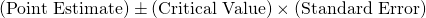Most CSU intro statistics courses will only cover the following confidence interval formulas:

Confidence IntervalFormula

CI for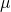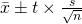CI forCI for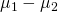(unpooled)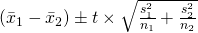CI for(pooled)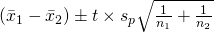CI for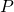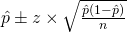CI for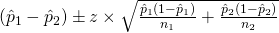### Example

EX: A random sample of 110 lightning flashes in a certain region resulted in a sample average radar echo duration of .81 sec and a sample standard deviation of .34 sec (“Lightning Strikes to an Airplane in a Thunderstorm,” J. ofAircraft, 1984: 607–611). Calculate a 95% (two-sided) confidence interval for the true average echo duration, and interpret the resulting interval.

#### Creating the CI

The first step to solving a CI problem is to figure out what CI formula we have to use. In order to do that, we carefully read the problem to find what kind of sample is being used and what the parameter of interest is. This problem involves one unpaired sample and explicitly tells us to create a CI for. As a result, the formula we have use is:The next step is to find the statistics required for our formula. Some questions will have these statistics in the context of the problem. By reading the example, we see that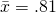,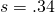, and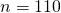. Other questions will provide a data set so we are able to calculate the statistics. Finding the critical value (CV) will depend on the course being taken. Some courses will approximate the CV and will let you know what value to use depending on the confidence. Otherwise, we will have to find the CV using either a Z or T table. The approximate CV to use for a 95% CI is 2. The correct CV to use that is found with a T table is 1.98.

Now that we have all the statistics, the final step is to plug and chug!We than have: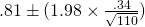We do our calculations and write the CI in the form of: (Lower Bound, Upper Bound)
So the 95% CI is: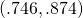#### Interpreting a CI

The last part of the question asks us to interpret the CI. Most interpretations will be very similar, but does require reading over the context of the problem. A general formula to follow would be:
We are ___ confident that the true mean ___ is between ___ and ___.

In the context of this problem it will be:
We are 95% confident that the true mean radar echo duration is between .746 seconds and .874 seconds.